• JAVA中数组作为函数参数传递 JAVA中数组作为函数参数传递： 在程序中，调用方法并且把数组的名称作为参数传递到方法中。 本质上是传递数组的地址值。 既然传递的是数组的地址，那么方法就可以通过数组的地址改变...
JAVA中数组作为函数参数传递
JAVA中数组作为函数参数传递： 在程序中，调用方法并且把数组的名称作为参数传递到方法中。 本质上是传递数组的地址值。 既然传递的是数组的地址，那么方法就可以通过数组的地址改变内存中数组的内容。 类似于C语言中调用函数传递数组的指针一样。可以在函数中通过指针改变数组的内容。 所以在JAVA当中，数组为引用类型，可以把数组作为参数传递到方法中去改变数组。例子：
public static void main(String[] args) {
int[] arr = {5,6,4,8,12,1,9,5};
sort(arr);
for(int i:arr)
System.out.print(i+" ");	//1 6 4 8 12 1 9 5
}

private static void sort(int[] a) {
a=1;
}

展开全文• 1 数组作为参数 我们可以将数组作为参数，传入到函数中，其实就...就是用数组作为函数参数； 又如， [java] view plain copy public class ArrayPar { public static void printArray(int []
1 数组作为参数
我们可以将数组作为参数，传入到函数中，其实就像我们main函数中 public void main(String [] args){};就是用数组作为函数参数；
又如，

[java]
view plain
copypublic class ArrayPar  {      public static void printArray(int [] array)      {          for(int i=0;i<array.length;i++)               System.out.print(array[i]+"  ");      }  }
我们可以这样调用 ArrayPar.printt(new int[ ]{3,2, 5,67});调用数组
这里的new int[ ]{3,2,5,67};他也是一种创建数组的方法，只是这种方法创建出来的数组是没有名字的，所以叫匿名数组。很多时候在只使用一次的时候可以使用匿名数组的方法法，类似的还有匿名类。
Java uses pass-by-value to pass arguments to a method. There are important differences between passing the values of variables of primitive data types and passing arrays. ■ For an argument of a primitive type, the argument’s value is passed. ■ For an argument of an array type, the value of the argument is a reference to an array; this reference value is passed to the method. Semantically, it can be best described as pass-by-sharing, i.e., the array in the method is the same as the array being passed. So if you change the array in the method, you will see the change outside the method.
java 使用值传参（pass_by_value）的方式来传递函数参数，只是值传递方式在处理原始数据类型参数与引用类型参数时候有不同，如果一个参数是原始数据类型，那么参数变量的值传递进去。如果是引用类型，是传进了引用变量的值（也就是说，只是将指向数据的引用的值给传进去了，也就是被调用的函数新建的空间放的是这个引用的值，那么也就是也指向了数组存在的内存），所以同样是值传递，引用类型的传入的当然是引用变量的值，指向了同一数组，那么函数内对数组进行的修改在函数退出后依旧是有效的。
例子：

[java]
view plain
copypublic class ArrayPar  {      public static void main(String [] args)      {          int x=1;          int y[]={1,2,3};          change(x,y);          System.out.println("x="+x+",y="+y);      }      public static void change(int num,int [] array)      {          num=100;          array=100;      }  }

图形表示：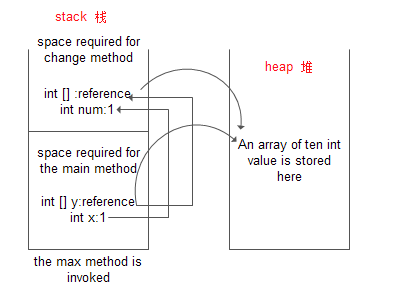这里同时注意一下，当我们用new 以及malloc这些的内存空间是在堆上heap，而像我们被调用的函数中使用的这些变量等在栈上。在调用changes时候，x的值被传入，在被调用的函数中重新开辟一个空间来放这个基本数据类型参数，但是int [ ] y ，将y传入其实就是传入了引用，在被调用的函数的栈上只会开辟一个空间来存放这个引用，所以被调用的函数与调用者 中两个引用指向堆上同一块内存。

2 数组做为函数返回值

[java]
view plain
copypublic static<span style="color:#ff0000;"> int []</span> reverse(int [] array)  {      int [] result=new int[array.length]      for(int i=0;i<array.length;i++)      {          result[i]=array[lenght-i];      }      return result;}
在将数组作为函数返回值时候如上红色标出的，就是在函数名字前加上返回值类型是int [ ] 表示返回一个int型数组，在函数体内最后返回是result这样的函数引用。

Case Study: Counting the Occurrences of Each Letter
write a program to count the occurrences of each letter in an random array of  lower  characters.
那么我们可以怎么做呢？
1）首先是要产生一个随机char数组  creatArray();（是否记得前边说过产生[a,a+b)之间的一个随机数 为  a+Math.random()*b,是否记得我们创建过获取任意字符的一个类？）
2) 创建一个数组 int [ ] count,长度26,准备来存放各个字母的计数
3）对数组进行循环 ， 每读取一个字母ch，则 count[ch-'a']++;

[java]
view plain
copyclass RandomChar  {      public static char getRandomChar(char ch1,char ch2)      {          return (char)(ch1+Math.random()*(ch2-ch1+1));      }      public static char getLowerCaseLetter()      {          return getRandomChar('a','z');      }      public static char getUpperCaseLetter()      {          return getRandomChar('A','Z');      }      public static char getDigitalLetter()      {          return getRandomChar('0','9');      }      public static char getRandomLetter()      {          return getRandomChar('\u0000','\uFFFF');      }  }  public class CountOccur  {      public static void main(String [] args)      {           char [] array=CreateArray();           int [] count = new int;           for(int i=0;i<array.length;i++)           {               count[array[i]-'a']++;           }           for(int i=0;i<count.length;i++)           {              System.out.print((char)(i+'a')+": "+count[i]+"    ");              if((i+1)%10==0) System.out.println();           }                            }      //产生一个100个元素的小写随机数组      public static char[] CreateArray()      {          char [] a=new char;          for(int i=0;i<a.length;i++)          {              a[i]=RandomChar.getLowerCaseLetter();          }          return a;      }  }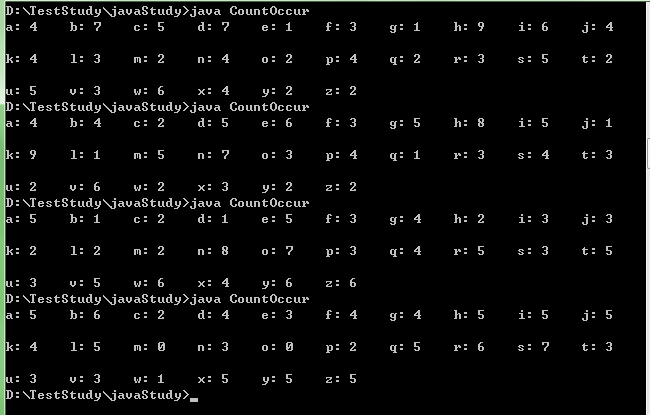3 可变长度参数列表
You can pass a variable number of arguments of the same type to a method. The parameter in the method is declared as follows: typeName... parameterName In the method declaration, you specify the type followed by an ellipsis Only one vari- able-length parameter may be specified in a method, and this parameter must be the last para- meter. Any regular parameters must precede it. Java treats a variable-length parameter as an array. You can pass an array or a variable number of arguments to a variable-length parameter. When invoking a method with a variable number of arguments, Java creates an array and passes the arguments to it
我们可以传递类型相同，但个数可以变化的参数到函数中，如果有这个需求的话，这时候我们只需要在形式参数中使用 typename...parameterName就可以达到这个目的，要注意，在这里声明的该变长参数必须是最后一个参数，任何常规参数必须在他之前，也就是说你可以有 MethodName(char b, double c, int ... nums) 这样的形式即int ... nums必须在最后一个位置，你不能将int ... nums 声明在参数参数列表的非最后位置。
java将可变长参数当做数组对待。可以将一个数组或者可的参数个数传递给该参数（注意，我们这里说该参数就是 typeName ... parameterName这整个结构），无论哪种形式，java会创建一个数组并把参数传给他，注意这里体会原文说的When invoking a method with a variable number of arguments， java Create an array and passes the arguments to it,也就是说，如果你是传入几个变长的变量，那么在调用时候java先将创建一个数组来装这几个实际参数，然后再执行调用的函数，如果本身我们传入一个数组，其实他并不会创建一个新的数组来装，还是一样像上面指向了已经分配的数组空间，所以在被调用的函数中对数组的改变在退出时候还是有效的（这是我用例子试了下体会到的）

[java]
view plain
copypublic class VariablePar  {      public static void main(String [] args)      {          int array[]={1,4,7,2,0};          System.out.println("max="+findMax(array));          ifChange(array);          System.out.println("array="+array);          ifChange(1,45,33);      }      public static int findMax(int ... nums)      {          if(nums.length==0)           {              System.out.println("No argumment passed");              return -1;          }          int max=nums;          for(int i=1;i<nums.length;i++)          {              if(max<nums[i]) max=nums[i];          }          return max;      }      //测试这里究竟是新创建一个数组还是相当于把引用传进来而已      public static void ifChange(int ... nums)      {          nums=100;      }  }
这里，我们从findMax中看到，他不用先说明 什么就可以直接使用nums.lenght 说明确实java是完全将这类型的参数当做一个数组来处理了，二在ifChange中，我们看到输出的是array=100,说明我们在调用ifChange(array)时候并不是重新创建一个新的数组，而是还是一样像前边的传入了引用，被调用者还是指向了相同的数组空间。但是在ifChage(1,45,33)这个调用时候，java就会先new int[ ]{1,45,33} 这样，然后形参nums再指向它，其实这样返回后应该就不会改变了1的值吧？
展开全文java 引用
• 文章目录一：数组元素作为函数的实参二：数组作为函数的实参关于数组作为函数参数调用的讨论关于函数定义的参数表的讨论获取二维数组的行和列更高维数组作为函数参数同二维数组类似参考文档 一：数组元素作为函数...
C语言数组作为函数参数

文章目录
一：数组元素作为函数的实参二：数组名作为函数的实参关于数组作为函数参数调用的讨论*将函数参数定义为指针的形式关于函数定义的参数表的讨论
三：二维数组名作为函数参数*将函数参数定义为指针的形式(1)第一维是指针，第二维是数组(2)二维指针并不能达到传递普通二维数组的效果(3)只有动态申请的二维数组才可通过二维指针作为函数参数传递
获取二维数组的行和列
四：更高维数组作为函数参数同二维数组类似五：参考文档

一：数组元素作为函数的实参
数组元素就是变量，与普通变量没有区别，将数组元素传送给形参，实现单向的值传递。 用数组元素作实参时，只要数组类型和函数的形参变量的类型一致，那么作为下标变量的数组元素的类型也和函数形参变量的类型是一致的。 因此，并不要求函数的形参也是下标变量。换句话说，对数组元素的处理是按普通变量对待的。
在普通变量或下标变量作函数参数时，形参变量和实参变量是由编译系统分配的两个不同的内存单元。在函数调用时发生的值传送是把实参变量的值赋予形参变量。
#include <stdio.h>
float max(float x,float y)
{
if(x > y)
return x;
else
return y;
}
int main()
{
int a = {3,2,1,4,9,0};
int m = a;
for(int i = 1;i < 6; i ++)
{
m = max(m,a[i]);
}
printf("数组中的最大元素是:%d",m);
}

二：数组名作为函数的实参
实质是地址的传递，将数组的首地址传给形参，形参和实参共用同一存储空间，形参的变化就是实参的变化。用数组名作函数参数时，则要求形参和相对应的实参都必须是类型相同的数组，都必须有明确的数组说明。当形参和实参二者不一致时，即会发生错误。在用数组名作函数参数时，不是进行值的传送，即不是把实参数组的每一个元素的值都赋予形参数组的各个元素。因为实际上形参数组并不存在，编译系统不为形参数组分配内存。
那么，数据的传送是如何实现的呢? 数组名就是数组的首地址, 因此在数组名作函数参数时所进行的传送只是地址的传送，也就是说把实参数组的首地址赋予形参数组名(既然如此，那函数参数自然可以为定义成指针的形式，后续会讲)。形参数组名取得该首地址之后，也就等于有了实在的数组。实际上是形参数组和实参数组为同一数组，共同拥有一段内存空间。
举例子：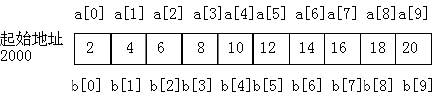上图说明了这种情形。图中设a为实参数组，类型为整型。a占有以2000为首地址的一块内存区。b为形参数组名。当发生函数调用时，进行地址传送，把实参数组a的首地址传送给形参数组名b，于是b也取得该地址2000。于是a，b两数组共同占有以2000为首地址的一段连续内存单元。从图中还可以看出a和b下标相同的元素实际上也占相同的两个内存单元（整型数组每个元素占二字节）。例如a和b都占用2000和2001单元，当然a等于b。类推则有a[i]等于b[i]。
举例说明，同时练习冒泡排序，小的数像水泡浮在上面。
#include <stdio.h>
//其中形参数组b没有给出长度，而由n值动态地表示数组的长度。n的值由主调函数的实参进行传送。
void sort(int b[], int n)
{
for (int i=0;i<n-1;i++)//n个数比较只需要比较n-1次，因为比较是两个数之间进行的，比如两个数比较只需要比较1次。
{
for (int j = 0; j < n - 1 - i; j++)
//可以这么理解当大的数沉底后，就不再参与排序了，循环1次，找出此轮中最大的数放在该轮比较的最底部，
//下一轮找出剩下数据中的最大值，并排到该轮最底部，排序了i次后，就有i个数退出排序，就只剩下n-1-i个数待排，这就是n-1-i的由来
{
if (b[j] > b[j + 1])
{
int temp = 0;
temp = b[j];
b[j] = b[j + 1];
b[j + 1] = temp;
}
}
}
}
int main()
{
int a = { 3,2,1,4,9,0 };
sort(&a, sizeof(a) / sizeof(a));
for (int i = 0; i < sizeof(a) / sizeof(a); i++)
{
printf("%d ", a[i]);
}
return 0;
}

关于数组作为函数参数调用的讨论
其中数组作为函数参数调用还可以写成如下形式：
sort(a, sizeof(a) / sizeof(a));
sort(&a, sizeof(a) / sizeof(a));

测试结果：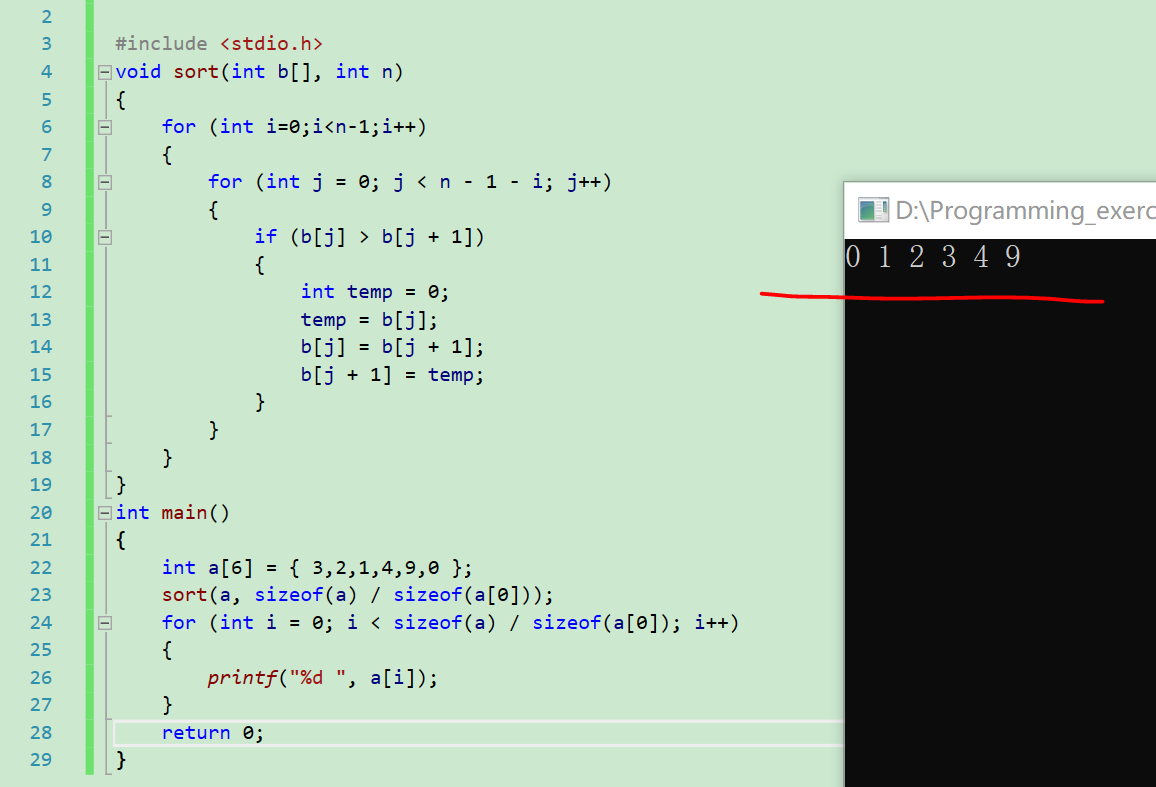*将函数参数定义为指针的形式
可以达到同样的效果 举例：
#include <stdio.h>
void sort(int* b, int n)
{
for (int i=0;i<n-1;i++)
{
for (int j = 0; j < n - 1 - i; j++)
{
if (b[j] > b[j + 1])
{
int temp = 0;
temp = b[j];
b[j] = b[j + 1];
b[j + 1] = temp;
}
}
}
}
int main()
{
int c = { 3,2,1,4,9,0 };
sort(c,6);
for (int i = 0; i < 6; i++)
{
printf("%d ", c[i]);
}
return 0;
}


测试结果：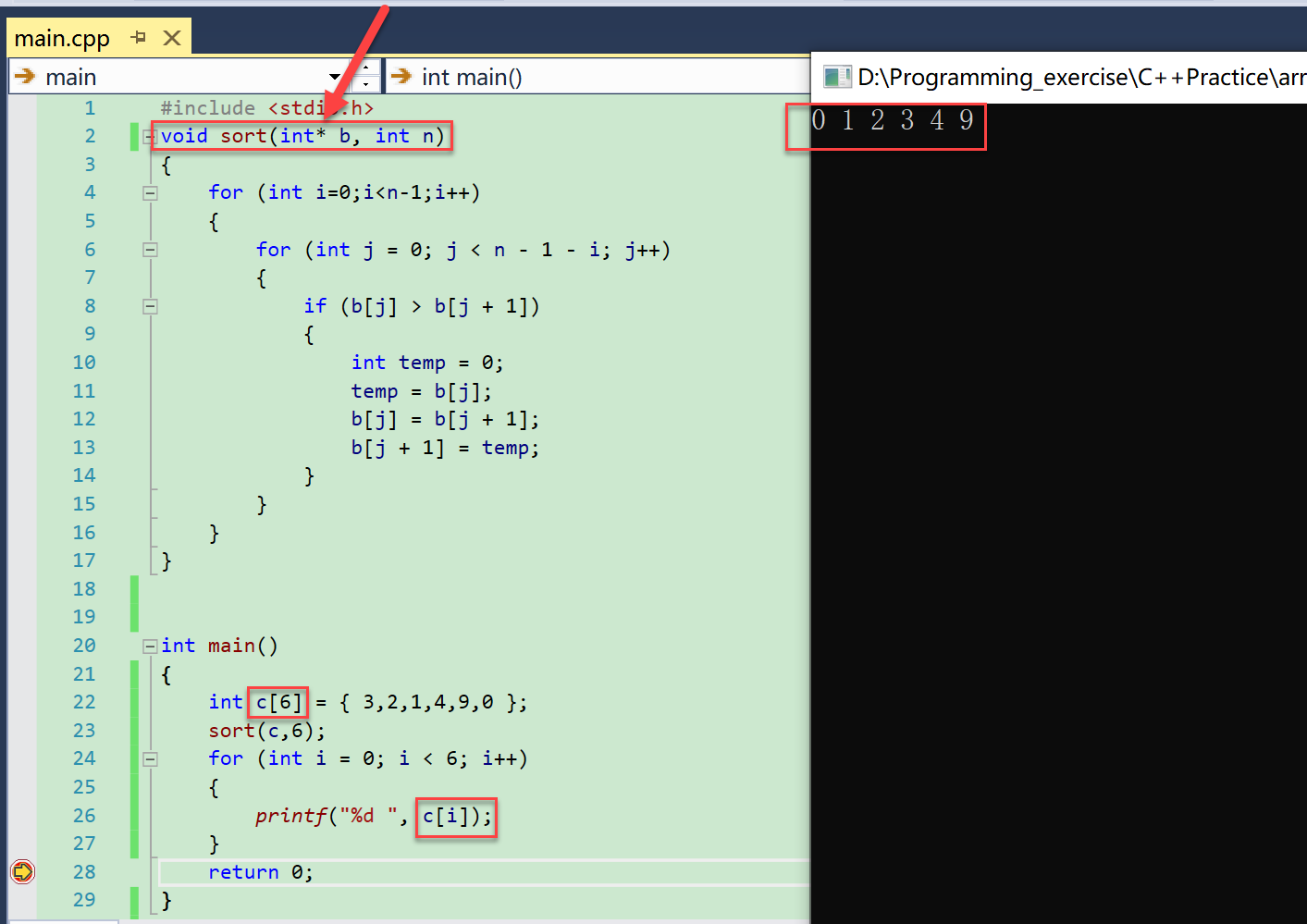关于函数定义的参数表的讨论
另外函数定义的参数表可以写成：
void sort(int b[], int n);
void sort(int b[x], int n);  //其中x≠0即可

其中x≠0即可，实际编程中写成这样也没必要，直接用void sort(int b[], int n)就行。 测试： x=1 小于a的数组长度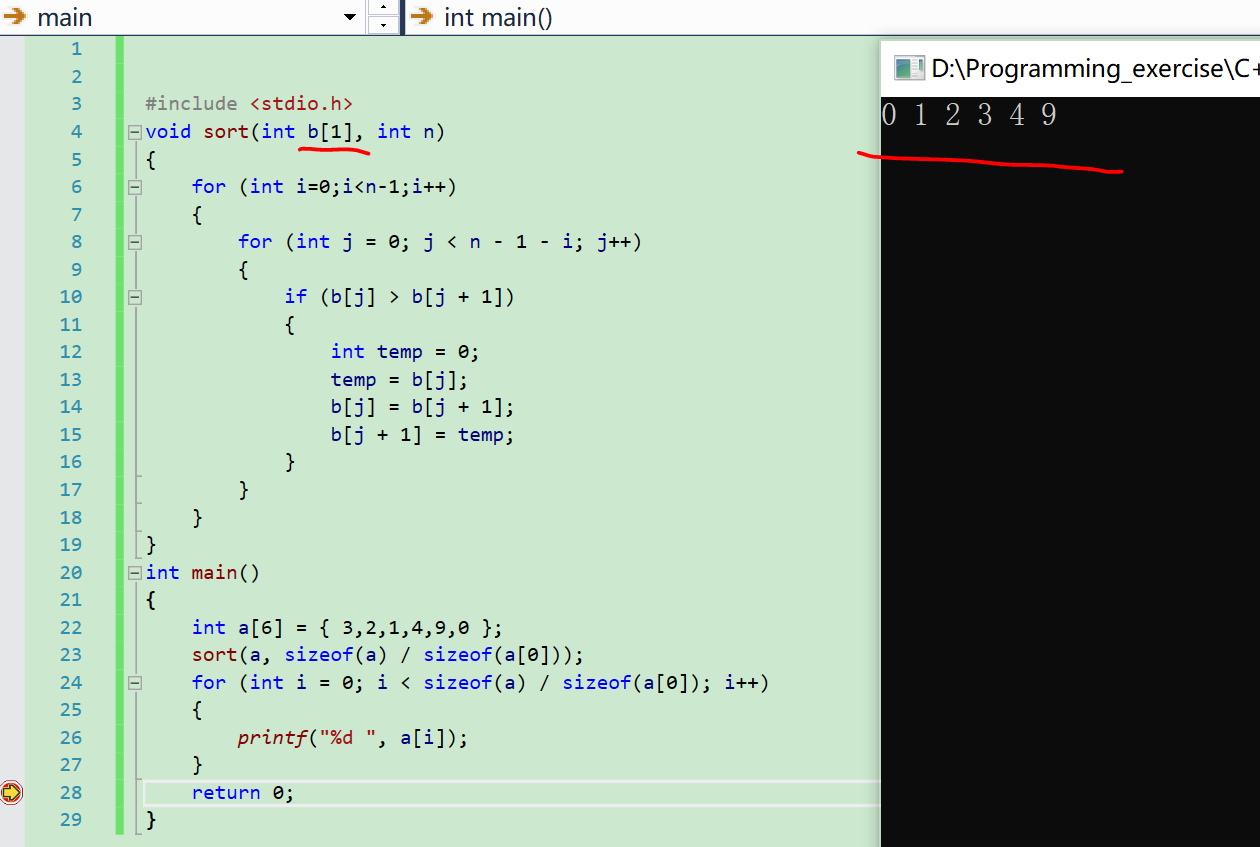x=100 大于a的数组长度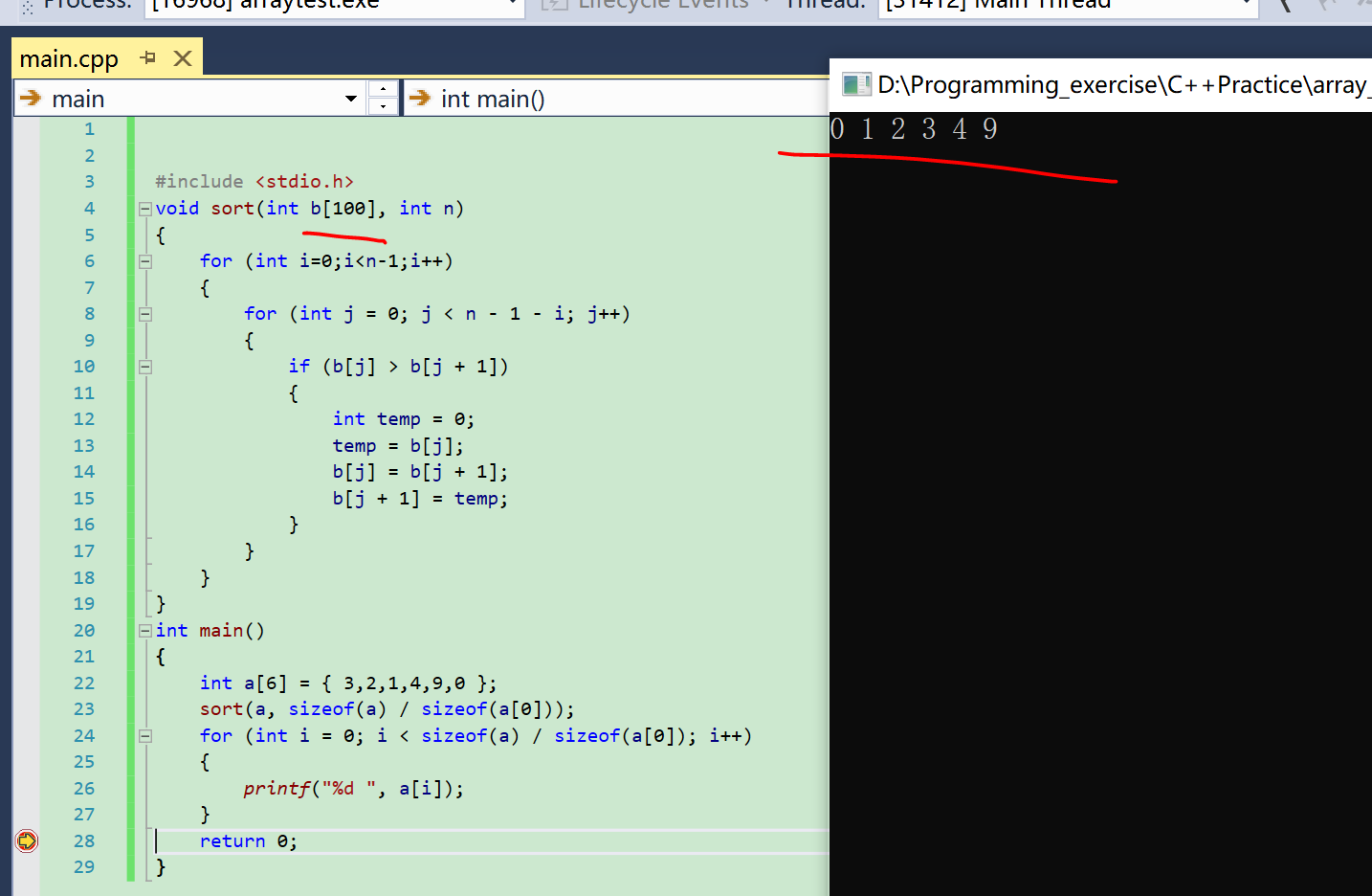但是当x为0时，编译都无法进行：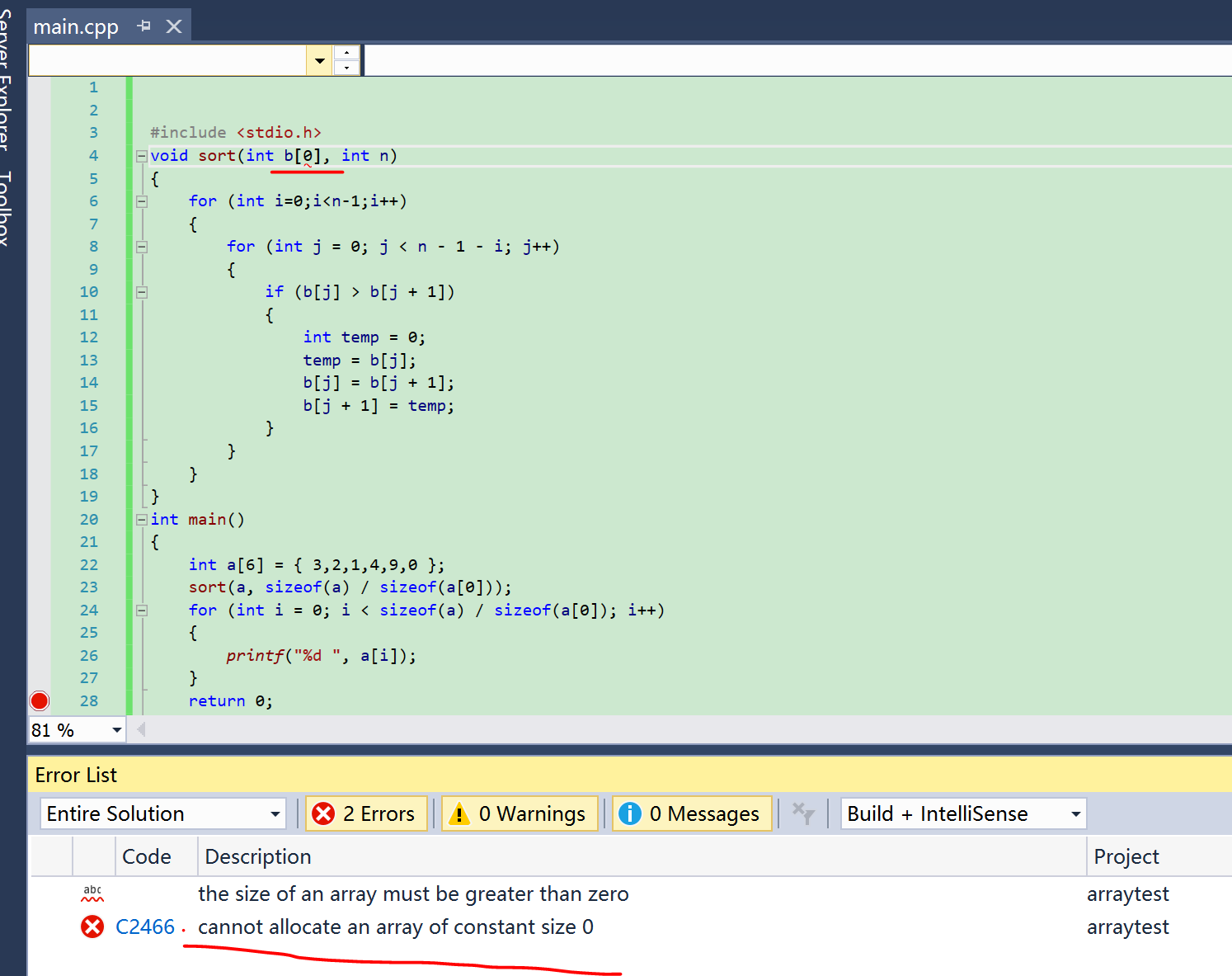三：二维数组名作为函数参数
第一维的大小可以不指定，第二维的大小必须指定。实参传送的是二维数组的首地址，使得二维数组a与b共用同一存储单元，即a与b共用同一存储单元，a与b共用同一存储单元。 测试：
#include <stdio.h>
//b也正确
int max(int b[])
{
int m = 0;
for (int i = 0; i < 2; i++)
{
for (int j = 0; j < 3; j++)
{
if (m < b[i][j])
{
m = b[i][j];
}
}
}
return m;
}

int main()
{
int a = { 3,2,1,4,9,0 };
int sizea = sizeof(a);
int maxVal = max(a);
printf("max is %d ", maxVal);
return 0;
}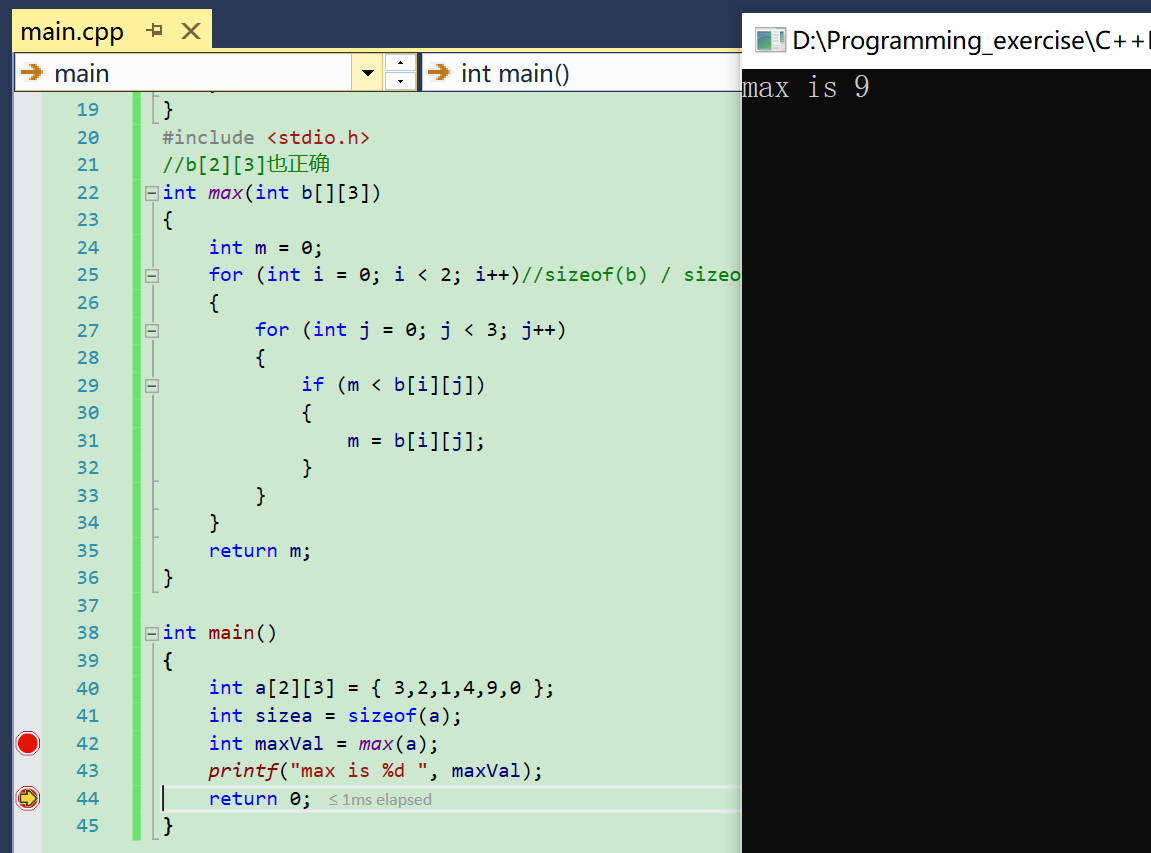注意形参二维数组的第二维必须与实参一致，否则会报错，如下图所示：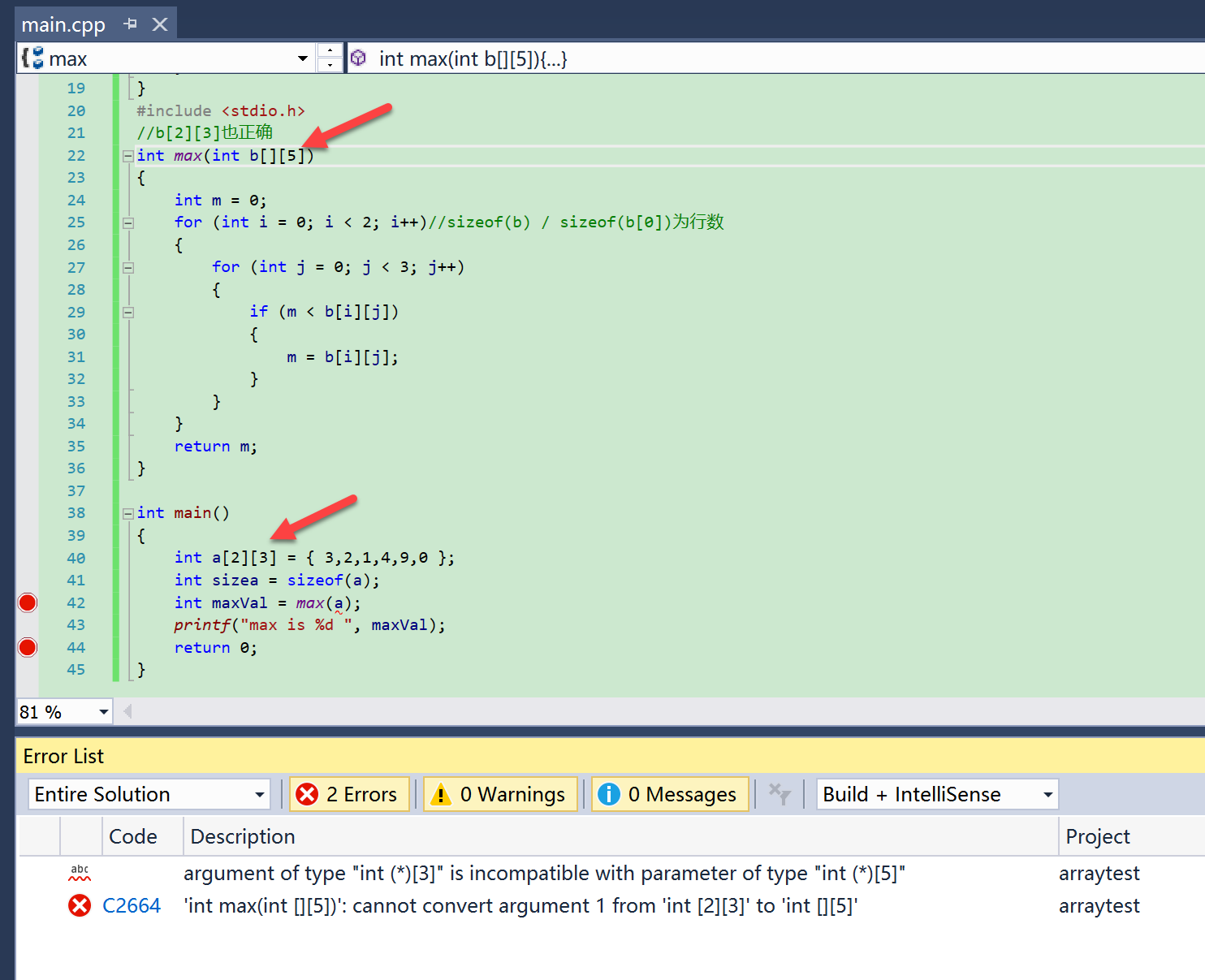第一维除了不能为0以外，都可以，实际编程中直接与实参的维度一样，没必要制造麻烦。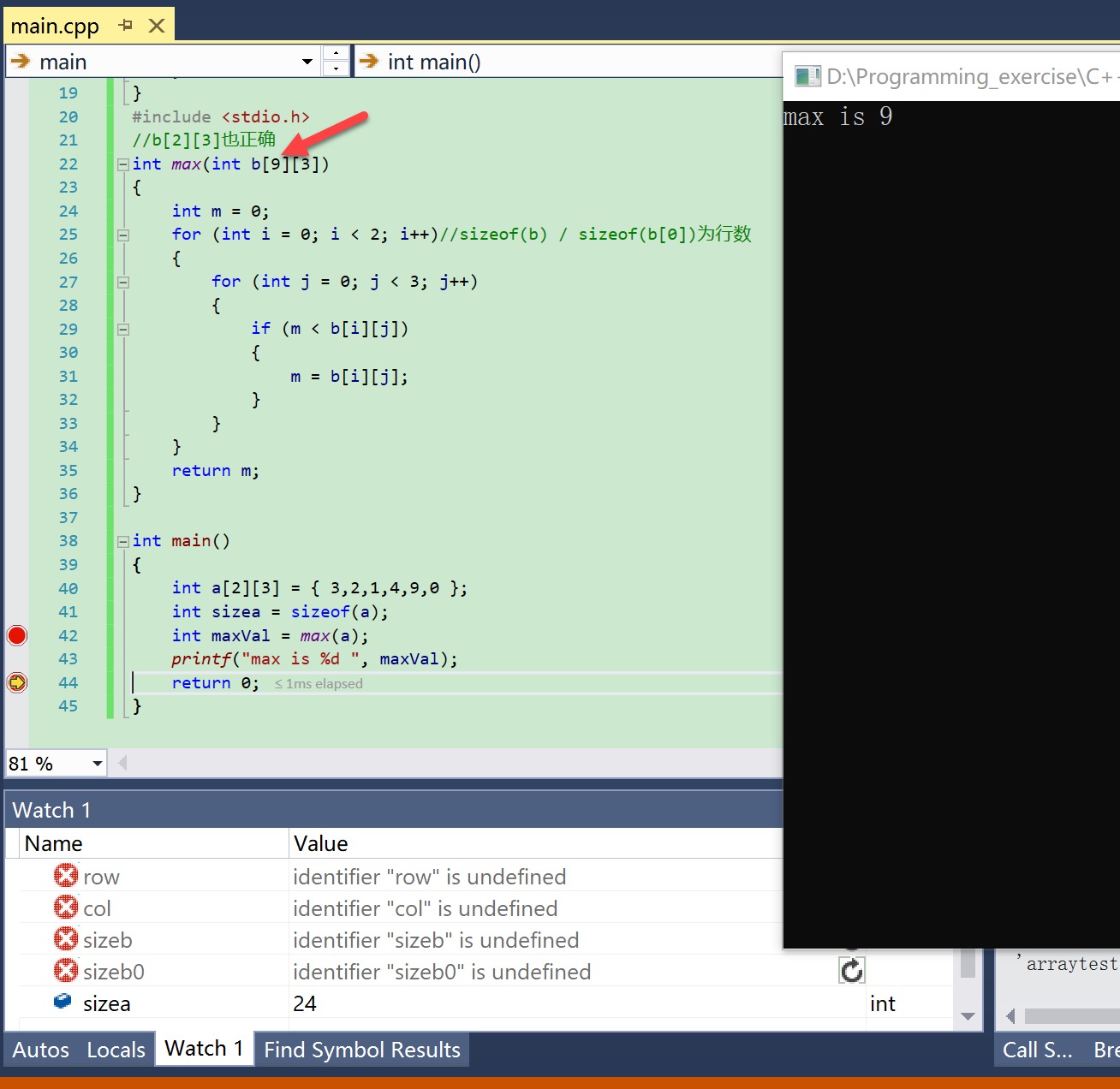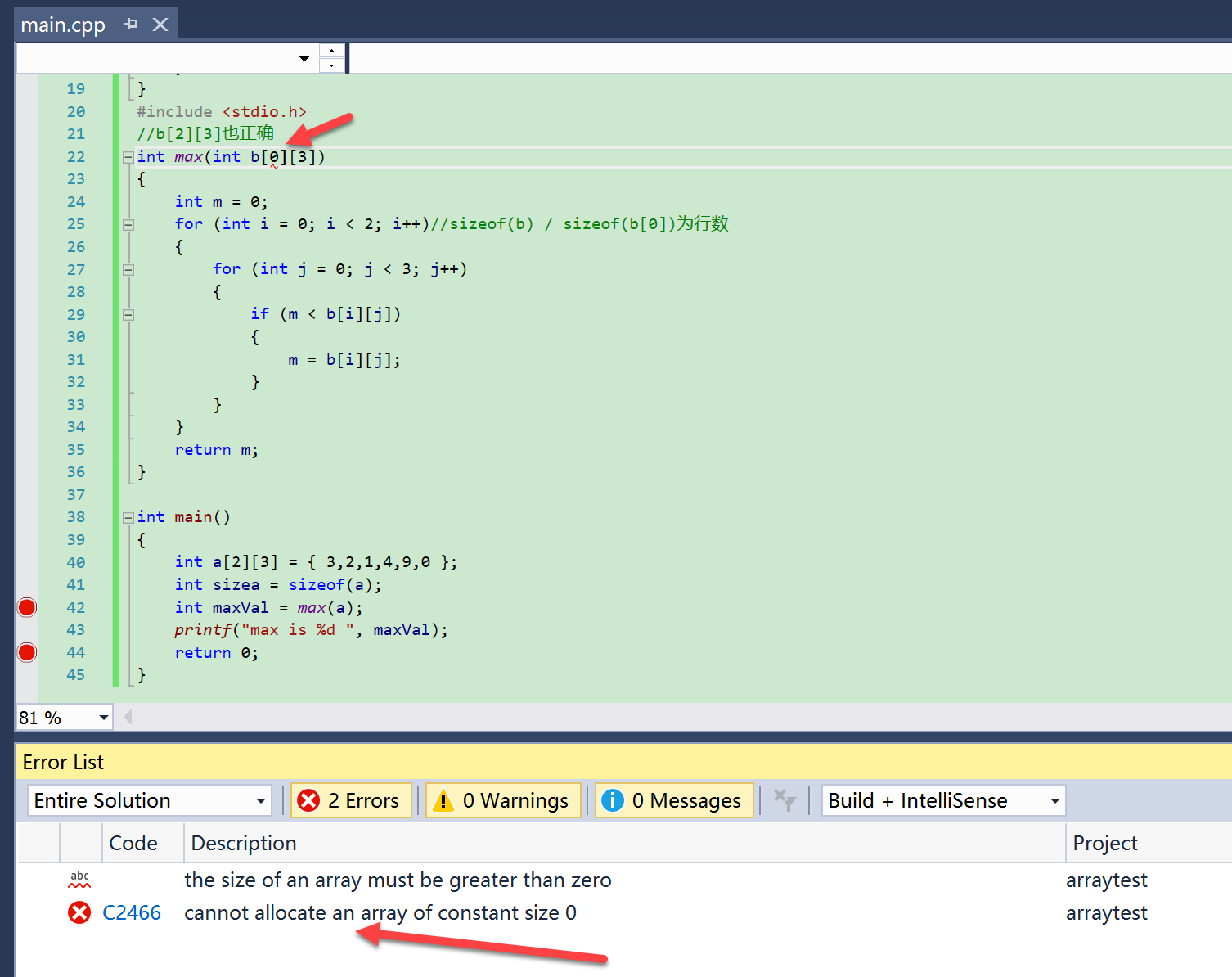*将函数参数定义为指针的形式
可以达到同样的效果
(1)第一维是指针，第二维是数组
且第二维数组的长度必须和实参的长度一样，否则调用该函数时编译无法通过
举例
#include <stdio.h>
int max(int (*b), int row, int col)
{
int m = 0;
for (int i = 0; i < row; i++)//sizeof(b) / sizeof(b)为行数
for (int j = 0; j < col; j++)
if (m < b[i][j])
m = b[i][j];

for (int i = 0; i < 2; i++)//sizeof(b) / sizeof(b)为行数
for (int j = 0; j < 3; j++)
b[i][j]=m;

return m;
}

int main()
{
int a = { 3,2,1,4,9,0 };
int maxVal = max(a,2,3);
printf("max is %d \n", maxVal);
for (int i = 0; i < 2; i++)
{
for (int j = 0; j < 3; j++)
{
printf("%d ", a[i][j]);
}
printf("\n");
}
return 0;
}

测试结果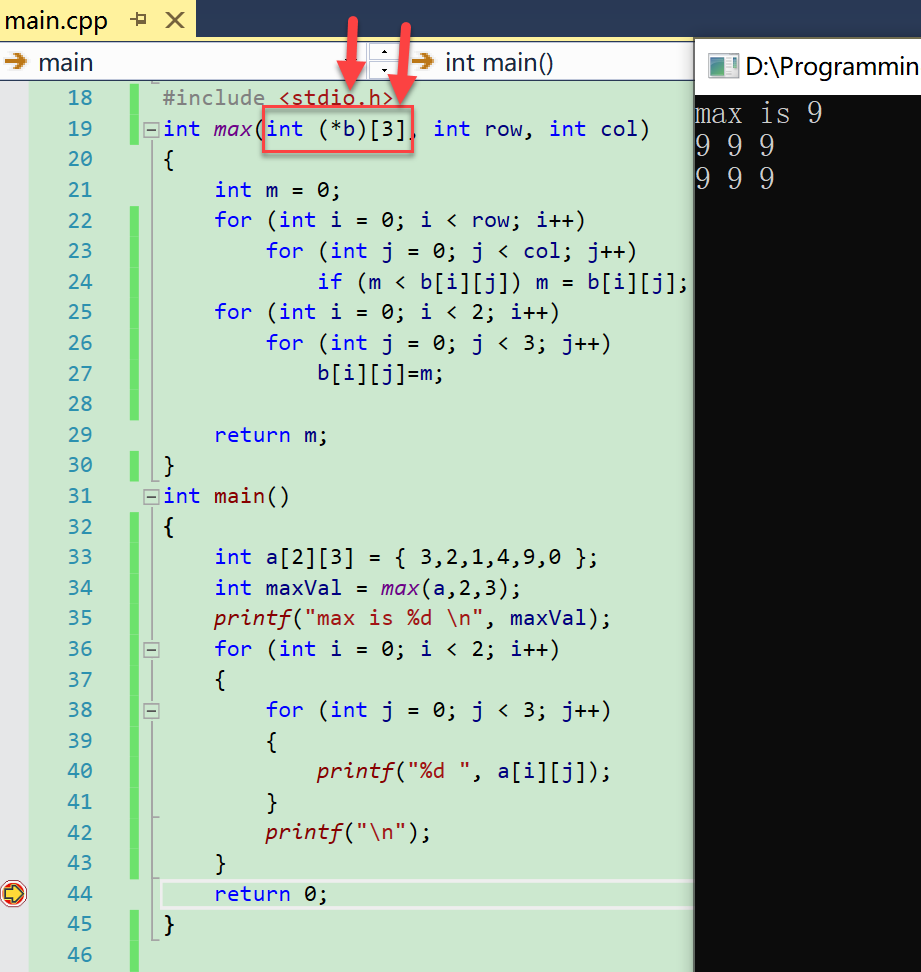(2)二维指针并不能达到传递普通二维数组的效果
现象：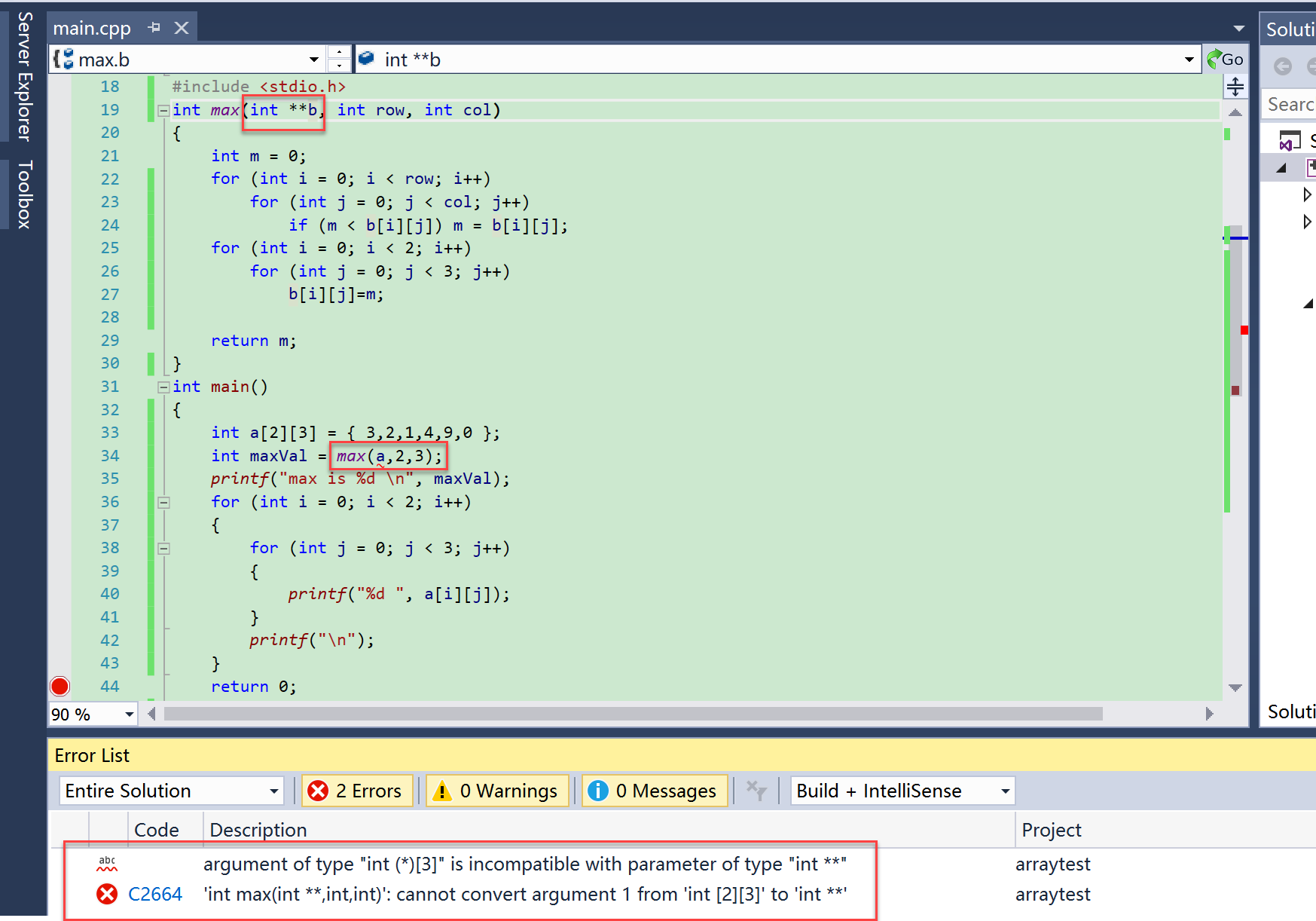强转也不行：原因 参考文档
(3)只有动态申请的二维数组才可通过二维指针作为函数参数传递
代码：
int max1(int **b, int row, int col)
{
int m = 0;
for (int i = 0; i < row; i++)
for (int j = 0; j < col; j++)
if (m < b[i][j]) m = b[i][j];
for (int i = 0; i < 2; i++)
for (int j = 0; j < 3; j++)
b[i][j] = m;

return m;
}
int main()
{
int a = { 3,2,1,4,9,0 };
int maxVal = max(a,2,3);
int row = 2,col = 3;
int** a1 = new int*[row];
for (int i = 0; i < row; i++)
a1[i] = new int[col];
for (int i = 0; i < row; i++)
for (int j = 0; j < col; j++)
a1[i][j] = (i+1)*j;
max1(a1,row,col);
for (int i = 0; i < 2; i++)
{
for (int j = 0; j < 3; j++)
{
printf("%d ", a1[i][j]);
}
printf("\n");
}
return 0;
}


测试：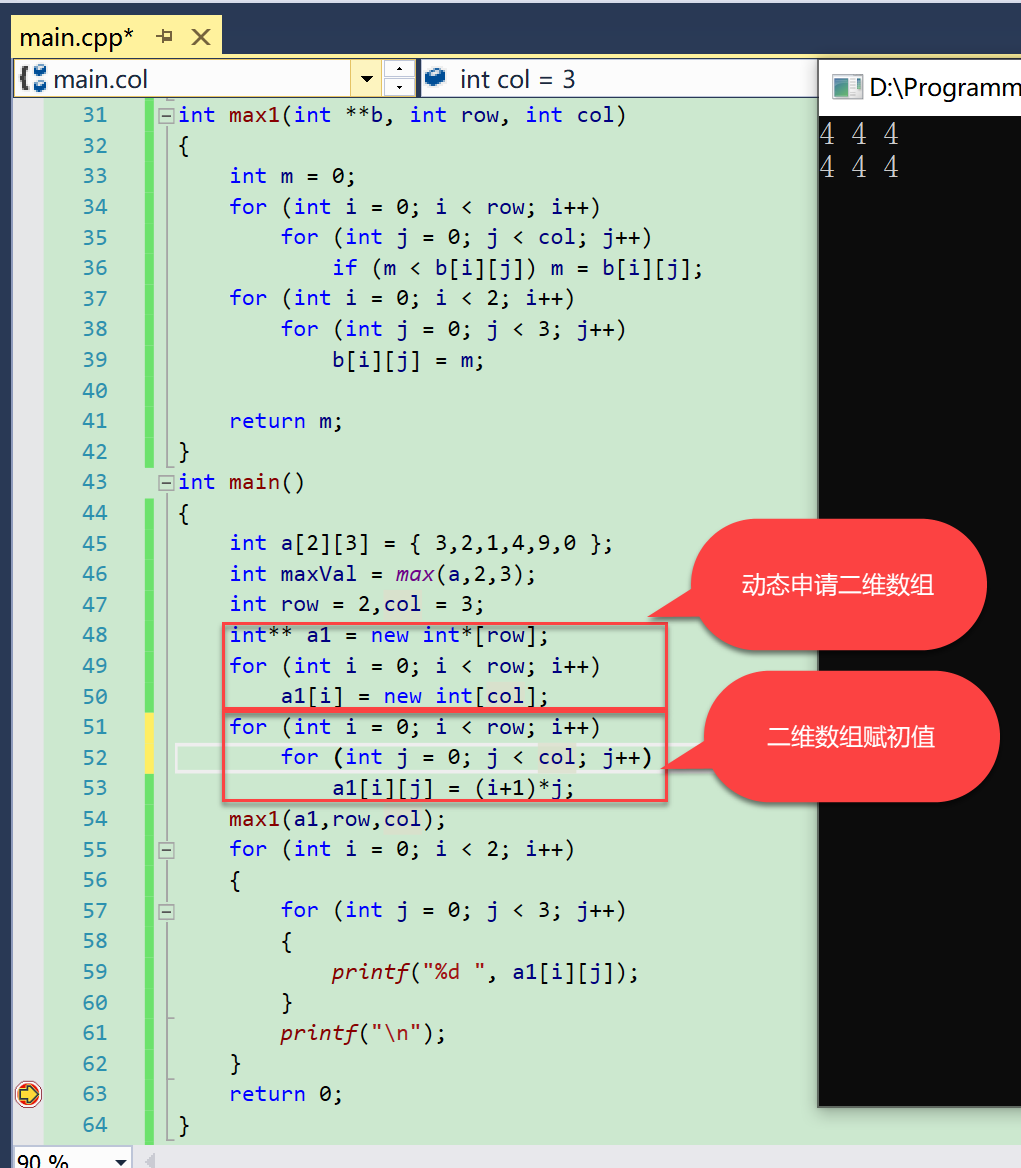获取二维数组的行和列
获取二维数组的行和列是经常遇到的

定义一个二维数组int array[A][B]，可以通过计算sizeof获取行列数。 sizeof(array)为一个元素占用的空间， sizeof(array)为一行元素占用的空间， sizeof(array)为整个数组占用的空间， 行数 = sizeof(array)/sizeof(array); 列数 = sizeof(array)/sizeof(array);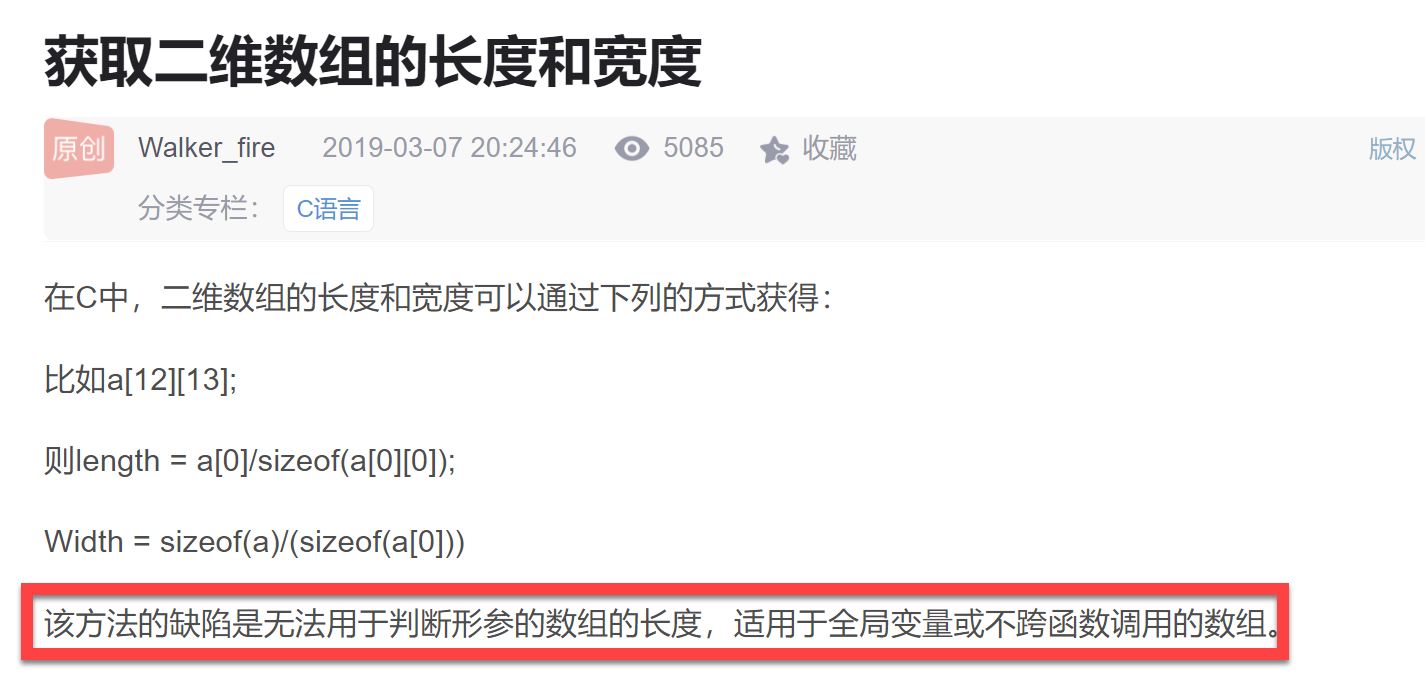根本原因：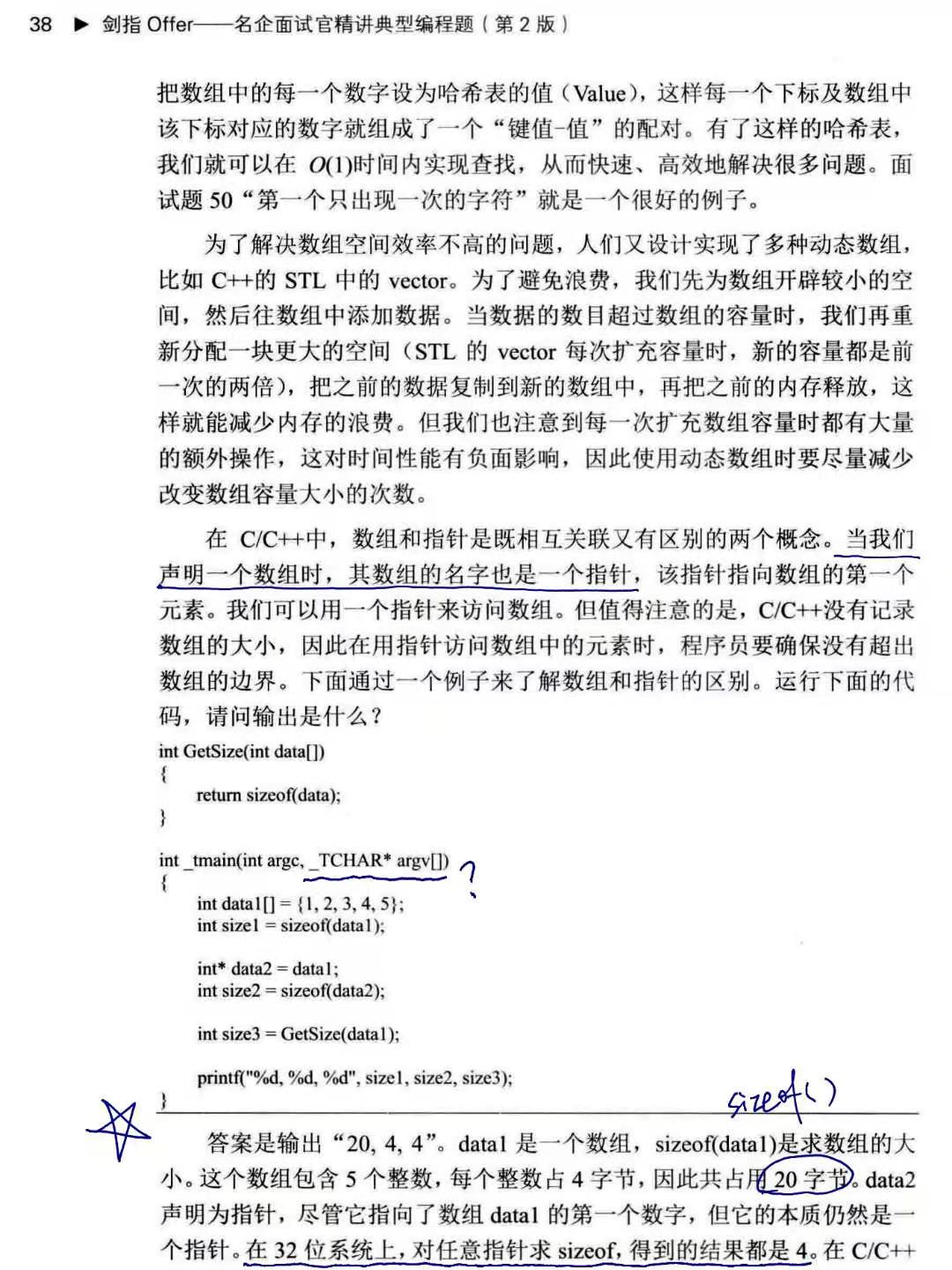测试：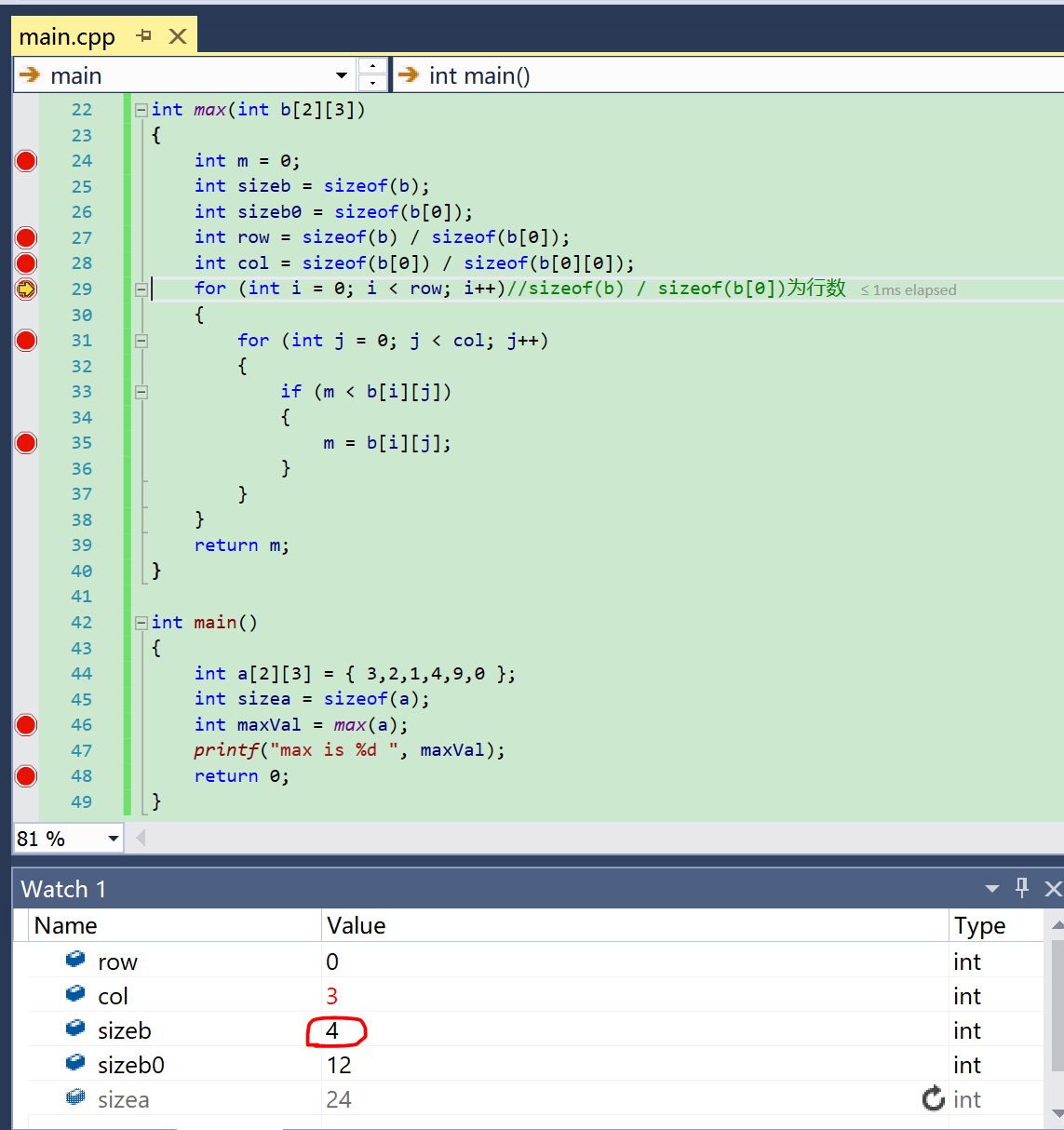四：更高维数组作为函数参数同二维数组类似
举例
#include "conv1.h"
#include <iostream>
#include <iomanip>
using namespace std;
float max(const float conv1_weight);
int main()
{
int a = { 0,1,2,
3,4,5 };
cout << a << endl;
cout << "numbers of conv1_weight: " << sizeof(conv1_weight) / sizeof(conv1_weight) << endl;
cout << "conv1_weight: " << setiosflags(ios::fixed) << setprecision(10) << conv1_weight << endl;
cout << "max of conv1_weight: " << max(conv1_weight) << endl;
int b = 16;
b >>= 1;
cout << b;
return 0;
}
float max(const float conv1_weight)
{
float max = 0;
for (int i = 0; i < 64; i++)
for (int j = 0; j < 3; j++)
for (int k = 0; k < 3; k++)
for (int m = 0; m < 3; m++)
for (int n = 0; n < 3; n++)
{
if (max < conv1_weight[i][j][k][m][n])
{
max = conv1_weight[i][j][k][m][n];
}
}
return max;
}

其中conv1_weight为下图所示：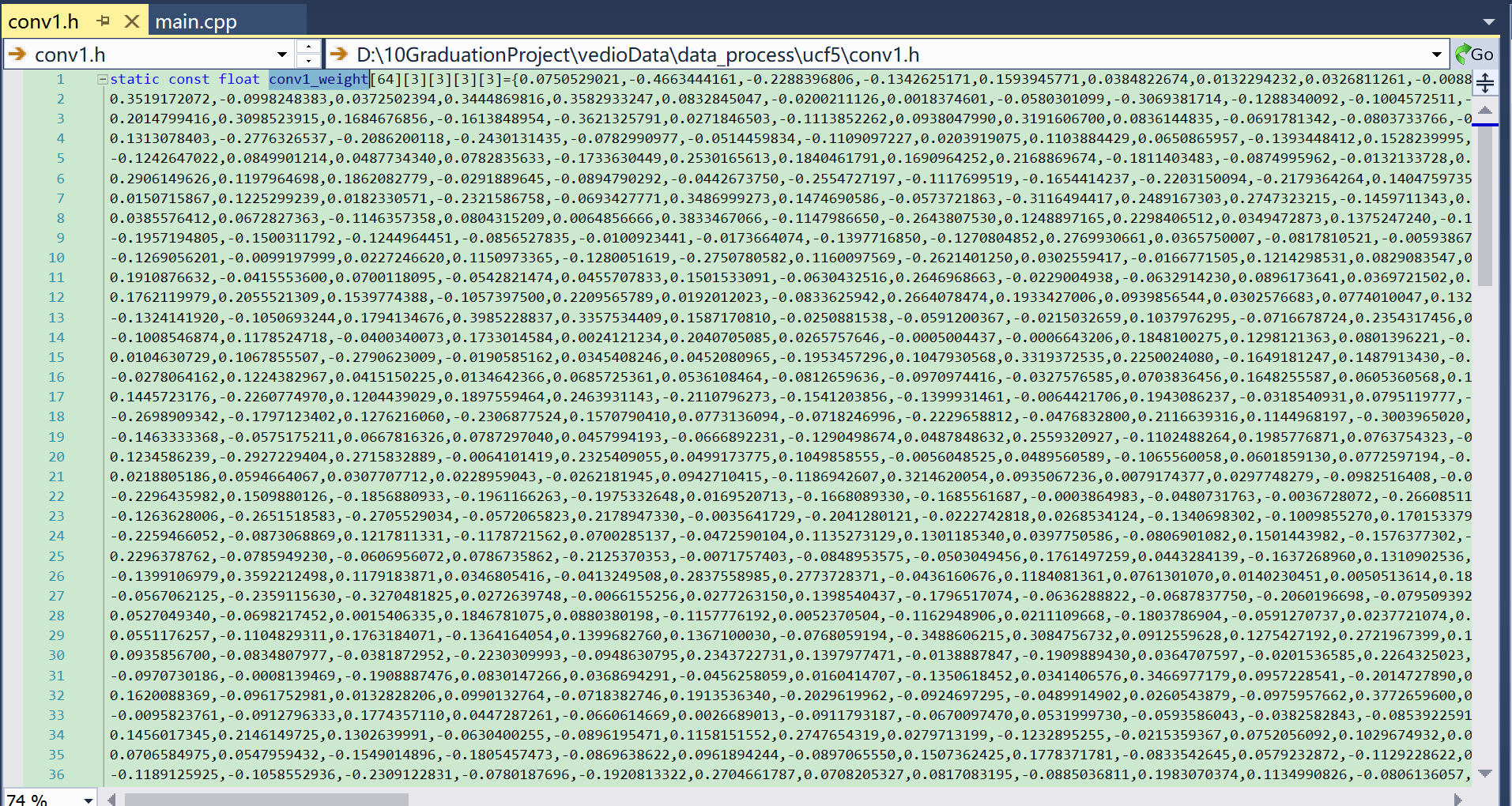测试结果：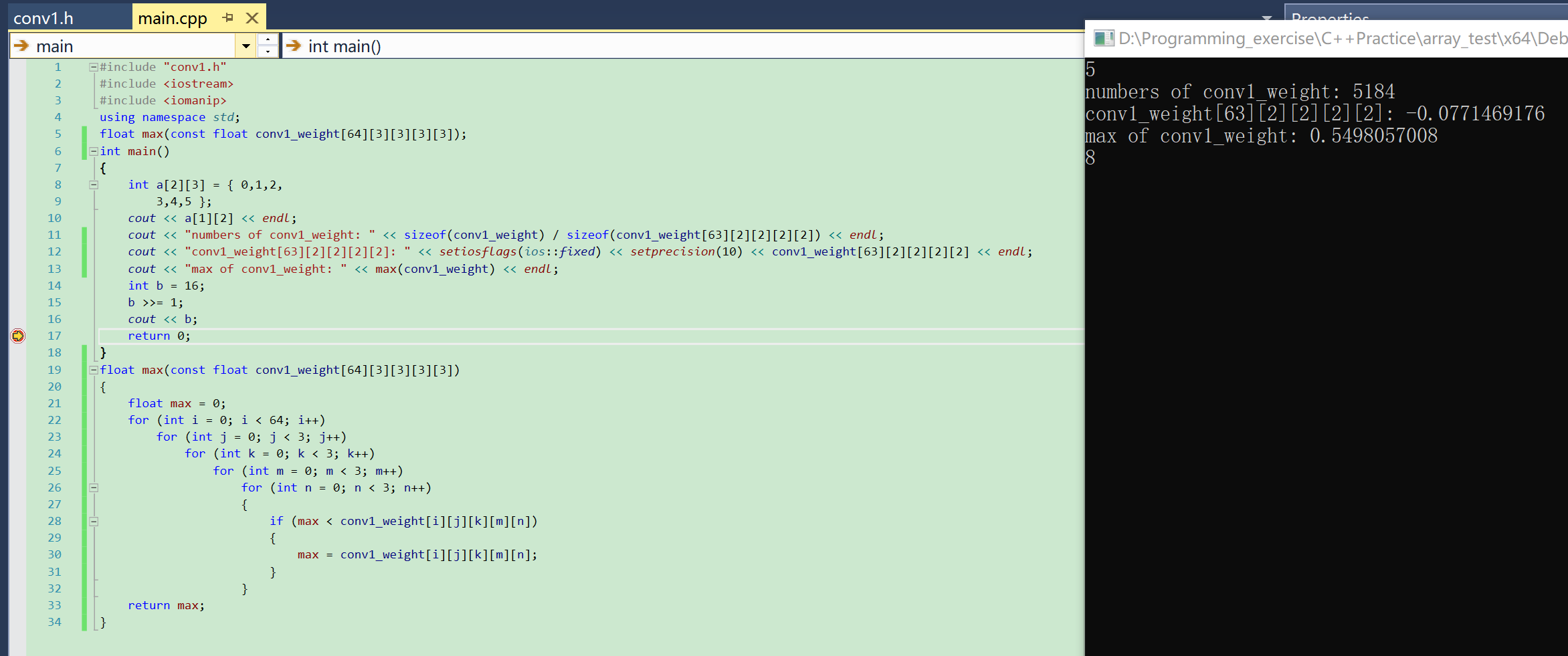五：参考文档
http://c.biancheng.net/cpp/html/61.html https://blog.csdn.net/lishundi/article/details/87906920
展开全文c++
• 然而，在将二维数组作为函数参数传递时，参数结构较复杂，难以理解。本文章是实用型文章，注重代码使用，不会讲述过多理论。如果想要学习理论知识（非常推荐，可以对代码的理解更透彻），可以查阅下方参考文献列出...
前言
多维数组中，二维数组是最常用的一种。在C语言编程中，二维数组的定义、取值以及赋值都比较容易，与一维数组类似。然而，在将二维数组作为函数参数传递时，参数结构较复杂，难以理解。本文章是实用型文章，注重代码使用，不会讲述过多理论。如果想要学习理论知识（非常推荐，可以对代码的理解更透彻），可以查阅下方参考文献列出书籍的第10章内容。话不多说，下面将给出一个C程序，以展示二维数组作为函数参数的4种方式。注：下面的代码已在VS Code(使用Mingw64)和VS 2015下编译运行过。
正文
下面程序的功能是对一个int型二维数组的所有元素进行求和，并分别把求和结果打印在屏幕上。根据二维数组传入函数的方式，定义了4个版本的求和函数。
#include <stdio.h>

#define ROW 2   //二维数组的行数
#define COL 2   //二维数组的列数

//4个版本的求和函数
//方式一：数组形式
int TwoDimArraySum1(int twoDimAr[][COL], int row, int col);

//方式二：指针形式，prArray是一个指向包含COL个int的数组的指针
int TwoDimArraySum2(int (*prArray)[COL], int row, int col);

//方式三：指针形式，pr是一个指向int的指针
int TwoDimArraySum3(int *pr, int row, int col);

//方式四：变长数组（C99开始支持）
int TwoDimArraySum4(int row, int col, int twoDimAr[row][col]);

int main(void)
{
int twoDimArray[ROW][COL] = {{-2, 5}, {4, 9}};
int result;

//方式一
result = TwoDimArraySum1(twoDimArray, ROW, COL);
printf("Sum1函数结果：%d\n", result);

//方式二
result = TwoDimArraySum2(twoDimArray, ROW, COL);
printf("Sum2函数结果：%d\n", result);

//方式三
result = TwoDimArraySum3(twoDimArray, ROW, COL);
printf("Sum3函数结果：%d\n", result);

//方式四
result = TwoDimArraySum4(ROW, COL, twoDimArray);
printf("Sum4函数结果：%d\n", result);

return 0;
}

int TwoDimArraySum1(int twoDimAr[][COL], int row, int col)
{
int i, j;
int result = 0;

for (i = 0; i < row; i++)
{
for (j = 0; j < col; j++)
{
//下面两种寻址方式都行
result += twoDimAr[i][j];
// result += *(*(twoDimAr + i) + j);
}
}
return result;
}

int TwoDimArraySum2(int (*prArray)[COL], int row, int col)
{
int i, j;
int result = 0;

for (i = 0; i < row; i++)
{
for (j = 0; j < col; j++)
{
//下面两种寻址方式都行
result += prArray[i][j];
// result += *(*(prArray + i) + j);
}
}
return result;
}

int TwoDimArraySum3(int *pr, int row, int col)
{
int i, j;
int result = 0;

for (i = 0; i < row; i++)
{
for (j = 0; j < col; j++)
{
//下面两种寻址方式都行
result += pr[i*col + j];
// result += *(Pr + i*col + j);
}
}
return result;
}

int TwoDimArraySum4(int row, int col, int twoDimAr[row][col])
{
int i, j;
int result = 0;

for (int i = 0; i < row; i++)
{
for (int j = 0; j < col; j++)
{
//下面两种寻址方式都行
result += twoDimAr[i][j];
// result += *(*(prArray + i) + j);
}
}
return result;
}
正如程序注释所言，方式4（变长数组）是C99开始支持的，不是所有编译器都支持。例如，VS 2015就不支持该语法。因此，在VS 2015环境下运行时，方式4被注释了。下面给出VS Code（基于Mingw64）以及VS 2015环境下的运行结果。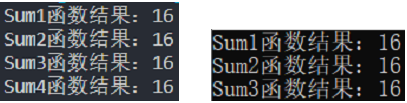左图为VS Code；右图为VS 2015
优缺点评价
这里先给出结论，最推荐使用方式3。下面是简要的分析。
方式1和方式2实质上是相同的。它们适用性很好。但是，它们定义必须事先给出第二维的长度，即不是对任意大小的二维数组都适用。方式3适用性同样很好，对任意大小的二维数组都适用。但是，它是最难理解的。理解它需要对二维数组元素的结构、二维数组元素的储存以及二维数组与指针的关系有比较深刻的理解。方式4是最容易理解的了。但是，它的适用性最差。
最后需要强调的是，对于该程序的求和函数，更安全、更易读的写法是将参数列表中接受二维数组数据的参数加上const修饰。本程序没加的原因是为了更好的突出其功能。const修饰的对象不同，产生的效果也不同。如果参数列表中接受二维数组数据的参数加上const修饰，它将无法修改二维数组的数据；如果只有待传入的二维数组是用const修饰，参数列表中的参数不是const修饰的，那么上述方式都不被允许。因此，请根据实际情况，自行决定const的修饰对象和修饰位置。
关于更高维数组
对于更高维的数组，上面四种方式仍然适用。除了方式3外，另外三种方式都很容易扩展成更高维的。对于方式3，虽然最推荐它，但是它随着维数的增多，它变得更复杂更难理解。而且基于方式3，还可以延伸出其他方式。这里以三维数组举例，方式3还可以延伸出这样一种方式，该方式中接受三维数组的形参是一个指向一维数组的指针。对于这种由方式3延伸的方式，我是不推荐的，它比方式3更复杂，使用它还不如使用方式3。
参考文献
Stephen Prata写的《C Primer Plus》第五版
博主：虔诚~似锦（主博客）
个性签名：如果你愿意努力，人生最坏的结果也不过是大器晚成。
------------------------------------------------------------------------------------
如果这篇文章对你帮助的话，记得在下方点赞哦，博主在此感谢！🎉🎉🎉
如果对这篇文章有疑问，请在评论区指出，欢迎探讨，共同进步。😄😄😄
展开全文c语言 vscode c++
• 一直以来弄不清C++中数组怎么作为参数传入函数中，尤其是最近学了Python之后，Python这种几乎可以把一切作为参数传入函数的方式更是让我在C++中混淆，下面来总结一下二维数组作为函数参数该如何表示。 1、二维数组的...
• C++中数组作为函数参数的注意问题 1 问题引入 从《剑指Offer》上的相关问题，下面的输出是什么？ #include<iostream> using namespace std; int GetSize(int data[]) { return sizeof(data); } int main() {...指针 c++
• 使用对象数组作为参数，只是将对象作为一个数组元素，其引用方法与基本数据类型的数组完全相同。但针对对象数组中的单个元素来讲，它又具有对象有属性和方法。 import java.util.Scanner; //学生类class Student{ ...
• ## 详谈C++中数组作为函数参数

万次阅读 多人点赞 2018-04-23 15:35:56
在C/C++中，当数组作为函数参数进行传递时，数组就自动退化为同类型的指针。因此尽管函数GetSize的参数data被声明为数组，但它会退化为指针，size3的结果仍然是4. 二、数组的两个特殊性质 (1)不允许拷贝和赋值  ...
• 1.首先，数组作为函数参数和普通的参数是有区别的，我们知道普通的参数如同void insert(int a,int b)之类的传递分为值传递，指针传递，指针值的传递，引用传递，它们的参数的传递是将实参的值赋给形参。但是在数组中...c++
• 函数可以以任意深度的数组或者对象的值作为排序基数对数组或的元素进行排序。 代码如下： /** * 排序数组或者对象 * by Jinko * date -- * @param object 数组对象 * @param subkey 需要排序的子键, 该...
• 原来 ndarray 对象作为参数传入函数的时候，如果同时传入多个参数，这些数组可以被当成普通的数来计算，但前提是这些数组的维数都相同，或者是0维的数（也其实相当于任意维），如果维数不同是无法处理的。...numpy
• C++分配数组调用带参数的构造函数 #include <iostream> using namespace std; class CStudent { public: CStudent(int num):age(num){} int age; }; int main(int argc, char const *argv[]) { cout &...
• 一、对象作为函数参数 对象也可以作为函数参数传递给函数，其转递方法与传递其他类型的数据一样，可采用值传递和地址传递两种方法。 值传递时是把对象的拷贝而不是本身传递给函数函数中对参数对象的任何修改...
• 在翻看以前学习资料时，发现如果用数组作为形参，其大小[即sizeof(形参名)]与指针大小一样——大小为 4。在网上找到一篇写的很好，所以转载过来作为笔记。C++ 语法 基础
• 如果形参是数组,那么实参是数组对象. 例如: void fun(int a[],int count); 参数一:表示的是int 型数组 参数二:表示传入的数组的个数 调用函数: int a = {1,0,1}; fun(a,3) 这个时候 a 就是数组对象 重点: 数组...
• 转载：... 在用二维数组作为参数传递时容易出现Segmention Error。这是因为不能正确为二维数组中元素寻址的问题，正确的方法如下：[cpp] view plaincopy #include <stdlib.
• 本文用简单的例子说明了C语言中数组函数参数,数组名a与&a区别,数组名a的"数据类型"，以及编译器的不同，相应指针所占字节的不同导致程序的细微差别。C语言
• 如果函数参数数组，尤其是高维数组，那就必须要同时定义数组的数据类型和元素个数。之前都是得过且过的用全局变量common块蒙混过关，都是祖师们从fortran77年代遗留下来的老程序了，全局变量到处都是，程序可读性...
• 在C/C++中，当数组作为函数参数进行传递时，数组就自动退化为同类型的指针。因此尽管函数GetSize的参数data被声明为数组，但它会退化为指针，size3的结果仍然是4. 二、数组的两个特殊性质 (1)不允许拷贝和...
• 数组作为方法参数2. 数组作为方法返回值4. 断点调试总结end 前言 以前的方法中我们学习了方法的参数和返回值，但是使用的都是基本数据类型。那么作为引用类型的数组能否作为 方法的参数进行传递呢，当然是可以的...Java
• 二、数组的类型 三、函数的类型（* 核心） 一、对象的类型：接口 概述：在面向对象语言中，接口（Interfaces）是一个很重要的概念，它是对行为的抽象，而具体如何行动需要由类（classes）去实现（implements） 1...typescript javascript object array function
• 对象数组中的元素同样需要用构造函数初始化。具体哪些元素用哪些构造函数初始化，取决于定义数组时的写法。 #include<iostream> using namespace std; class CSample{ public: CSample(){ //构造函数 1 cout...c++
• 结论今天一反常态，先说结论——会！，探究这个问题的起因是工作中遇到了这个问题。本来记得new一个对象一般情况下会调用这个对象的构造函数，可是一时间记不清楚数组会不会调用了，于是决定试一下对象
• 用指针做参数 #include &lt;stdio.h&gt; void swap(int *a, int* b) { int t = *a; *a = *b; *b = t; } int main() { int a = 3, b = 4; swap(&amp;a, &amp;b); printf("%d %d",...
• 但是C++中关于构造函数参数对象数组的使用却不是那么友好 以下为对C++中关于构造函数参数对象数组的使用的几种方法 class Test { public: int id; Test(int i) { id = i; } ~Test() { ...C++
• //对象数组作为类的成员 } 那样的话对象数组的初始化会变得很麻烦， 因为数组名不能作为左值，所以不可以指针传递的方式赋值。 而且不能通过参数列表（构造函数后面加一个冒号）的方式初始化， 所以只能让类...c++
• 今天学习了一下部分 ES6的 数组函数对象，做个简单的分享。 数组的高阶方法 filter 过滤 forEach 遍历 映射 map reduce 累计 a，b两个参数 上一次计算结果是这次的a every 每个 some 有一个 数组的新增方法 1....js...